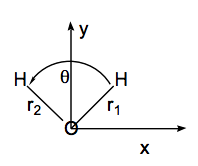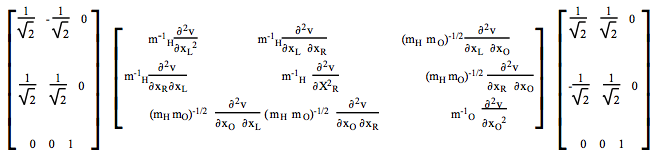Skip to main content

# 13.2: Vibrational Motion Within the Harmonic Approximation

•• Contributed by Jack Simons
• Professor Emeritus and Henry Eyring Scientist (Chemistry) at University of Utah

The simple harmonic motion of a diatomic molecule was treated in Chapter 1, and will not be repeated here. Instead, emphasis is placed on polyatomic molecules whose electronic energy's dependence on the 3N Cartesian coordinates of its N atoms can be written (approximately) in terms of a Taylor series expansion about a stable local minimum. We therefore assume that the molecule of interest exists in an electronic state for which the geometry being considered is stable (i.e., not subject to spontaneous geometrical distortion).

The Taylor series expansion of the electronic energy is written as:

$V(q_k) = V(0) + \sum\limits_k \left( \dfrac{\partial V}{\partial q_k} \right) q_k + \dfrac{1}{2}\sum\limits_{j,k}q_j H_{j,k} + ... ,$

where V(0) is the value of the electronic energy at the stable geometry under study, $$q_k$$ is the displacement of the $$k_{th}$$ Cartesian coordinate away from this starting position, $$\left( \frac{\partial V}{\partial q_k} \right)$$ is the gradient of the electronic energy along this direction, and the $$H_{j,k}$$ are the second derivative or Hessian matrix elements along these directions $$H_{j,k} = \left( \frac{\partial^2 V}{\partial q_j\partial q_k} \right).$$ If the starting geometry corresponds to a stable species, the gradient terms will all vanish (meaning this geometry corresponds to a minimum, maximum, or saddle point), and the Hessian matrix will possess 3N - 5 (for linear species) or 3N -6 (for non-linear molecules) positive eigenvalues and 5 or 6 zero eigenvalues (corresponding to 3 translational and 2 or 3 rotational motions of the molecule). If the Hessian has one negative eigenvalue, the geometry corresponds to a transition state (these situations are discussed in detail in Chapter 20).

From now on, we assume that the geometry under study corresponds to that of a stable minimum about which vibrational motion occurs. The treatment of unstable geometries is of great importance to chemistry, but this Chapter deals with vibrations of stable species. For a good treatment of situations under which geometrical instability is expected to occur, see Chapter 2 of the text Energetic Principles of Chemical Reactions by J. Simons. A discussion of how local minima and transition states are located on electronic energy surfaces is provided in Chapter 20 of the present text.

## The Newton Equations of Motion for Vibration

The Kinetic and Potential Energy Matrices

Truncating the Taylor series at the quadratic terms (assuming these terms dominate because only small displacements from the equilibrium geometry are of interest), one has the so-called harmonic potential:

$V(q_k) = V(0) + \dfrac{1}{2}\sum\limits_{j,k}q_j H_{j,k}q_k.$

The classical mechanical equations of motion for the 3N {$$q_k$$} coordinates can be written in terms of the above potential energy and the following kinetic energy function:

$T = \dfrac{1}{2}\sum\limits_jm_j\dot{q_j}^2,$

where $$\dot{q_j}$$ denotes the time rate of change of the coordinate $$q_j \text{ and } m_j$$ is the mass of the atom on which the $$j^{th}$$ Cartesian coordinate resides. The Newton equations thus obtained are:

$m_j \ddot{q_j} = - \sum\limits_k H_{j,k}q_k$

where the force along the $$j^{th}$$ coordinate is given by minus the derivative of the potential V along this coordinate $$\frac{\partial V}{\partial q_j}= \sum\limits_k H_{j,k}q_k$$ within the harmonic approximation.

These classical equations can more compactly be expressed in terms of the time evolution of a set of so-called mass weighted Cartesian coordinates defined as:

$x_j = q_j\sqrt{m_j},$

in terms of which the Newton equations become

$\ddot{x_j} = -\sum\limits_k H'_{j,k}x_k$

and the mass-weighted Hessian matrix elements are

$H'_{j,k} = H_{j,k}\dfrac{1}{\sqrt{m_jm_k}}.$

The Harmonic Vibrational Energies and Normal Mode Eigenvectors

Assuming that the $$x_j$$ undergo some form of sinusoidal time evolution:

$x_j(t) = x_j(0)cos(\omega t),$

and substituting this into the Newton equations produces a matrix eigenvalue equation:

$\omega^2x_j = \sum\limits_k H'_{j,k}x_k$

in which the eigenvalues are the squares of the so-called normal mode vibrational frequencies and the eigenvectors give the amplitudes of motion along each of the 3N mass weighted Cartesian coordinates that belong to each mode.

Within this harmonic treatment of vibrational motion, the total vibrational energy of the molecule is given as

$E(v_1, v_2, ... V_{3N-5 \text{ or }6}) = \sum\limits_{j=1}^{3N-5 \text{ or } 6} \hbar\omega_j \left( v_j + \dfrac{1}{2} \right)$

as a product of 3N-5 or 3N-6 harmonic oscillator functions $$\Psi_{vj}(x_j)$$ are for each normal mode within this picture, the energy gap between one vibrational level and another in which one of the $$v_j$$ quantum numbers is increased by unity (the origin of this "selection rule" is discussed in Chapter 15) is

$\Delta E_{vj} \rightarrow v_j + 1 = \hbar\omega_j$

The harmonic model thus predicts that the "fundamental" $$(v=0 \rightarrow v = 1) \text{ and "hot band" } (v=1 \rightarrow v = 2)$$ transition should occur at the same energy, and the overtone (v=0 Æ v=2) transitions should occur at exactly twice this energy.

## The Use of Symmetry

Symmetry Adapted Modes

It is often possible to simplify the calculation of the normal mode frequencies and eigenvectors by exploiting molecular point group symmetry. For molecules that possess symmetry, the electronic potential $$V(q_j)$$ displays symmetry with respect to displacements of symmetry equivalent Cartesian coordinates. For example, consider the water molecule at its $$C_{2v}$$ equilibrium geometry as illustrated in the figure below. A very small movement of the $$H_2O$$ molecule's left H atom in the positive x direction $$(\Delta x_L)$$ produces the same change in V as a correspondingly small displacement of the right H atom in the negative x direction $$(-\Delta x_R).$$ Similarly, movement of the left H in the positive y direction $$(\Delta y_L)$$ produces an energy change identical to movement of the right H in the positive y direction $$(\Delta y_R).$$Figure 13.2.1: Insert caption here!

The equivalence of the pairs of Cartesian coordinate displacements is a result of the fact that the displacement vectors are connected by the point group operations of the $$C_{2v}$$ group. In particular, reflection of $$\Delta x_L$$ through the yz plane produces $$-\Delta x_R$$, and reflection of $$\Delta y_L$$ through this same plane yields $$\Delta y_R.$$

More generally, it is possible to combine sets of Cartesian displacement coordinates {$$q_k$$} into so-called symmetry adapted coordinates {$$Q_{Gamma,j}$$}, where the index $$\Gamma$$ labels the irreducible representation and j labels the particular combination of that symmetry. These symmetry adapted coordinates can be formed by applying the point group projection operators to the individual Cartesian displacement coordinates.

To illustrate, again consider the $$H_2O$$ molecule in the coordinate system described above. The 3N = 9 mass weighted Cartesian displacement coordinates $$(X_L, Y_L, Z_L, X_O, Y_O, Z_O, X_R, Y_R, Z_R)$$ can be symmetry adapted by applying the following four projection operators:

$P_{A_1} = 1 + \sigma_{yz} + \sigma_{xy} + C_2$

$P_{b_1} = 1 + \sigma_{yz} + \sigma_{xy} - C_2$

$P_{b_2} = 1 - \sigma_{yz} + \sigma_{xy} - C_2$

$P_{a_2} = 1 - \sigma_{yz} - \sigma_{xy} + C_2$

to each of the 9 original coordinates. Of course, one will not obtain 9 x 4 = 36 independent symmetry adapted coordinates in this manner; many identical combinations will arise, and only 9 will be independent.

The independent combination of $$\textbf{ a_1 symmetry } (normalized to produce vectors of unit length) are $Q_{a_1,1} = \dfrac{1}{\sqrt{2}}[X_L - X_R]$ $Q_{a_1,2} = \dfrac{1}{\sqrt{2}}[Y_L - Y_R]$ $Q_{a_1,3} = [Y_O]$ Those of \(b_2$$ symmetry are

$Q_{b_2,1} = \dfrac{1}{\sqrt{2}}[X_L + X_R]$

$Q_{b_2,2} = \dfrac{1}{\sqrt{2}}[Y_L - Y_R]$

$Q_{b_2,3} = [X_O]$

and the combinations

$Q_{b_1,1} = \dfrac{1}{\sqrt{2}}[Z_L + Z_R]$

$Q_{b_1,2} = [Z_O]$

are of $$b_1$$ symmetry, whereas

$Q_{a_2,1} = \dfrac{1}{\sqrt{2}}[Z_L - Z_R]$

is of $$a_2$$ symmetry.

Point Group Symmetry of the Harmonic Potential

These nine $$Q_{\Gamma,j}$$ are expressed as unitary transformations of the original mass weighted Cartessian coordinates:

$Q_{\Gamma,j} = \sum\limits_k C_{\Gamma, j, k}X_k$

These transformation coefficients {$$C_{\Gamma, j, k}$$} can be used to carry out a unitary transformation of the 9x9 mass-weighted Hessian matrix. In so doing, we need only form blocks

$H^{\Gamma}_{j,1} = _{k k'}C_{\Gamma , j, k}H_{k, k'}\dfrac{1}{\sqrt{m_k m_k'}}C_{\Gamma , 1, k'}$

within which the symmetries of the two modes are identical. The off-diagonal elements

$H_{j 1}^{\Gamma \Gamma '} = _{k k'}C_{\Gamma , j, k}H_{k, k'}\dfrac{1}{\sqrt{m_km_k'}}C_{\Gamma ' , 1, k'}$

vanish because the potential $$V(q_j) \text{ (and the full vibrational Hamiltonian H = T + V) commutes with the }C_{2V}$$ point group symmetry operations.

As a result, the 9x9 mass-weighted Hessian eigenvalue problem can be sub divided into two 3x3 matrix problems ( of $$a_1 \text{ and } b_2$$ symmetry), one 2x2 matrix of $$b_1 \text{ symmetry and one 1x1 matrix of } a_2 \text{ symmetry. For example, the } a_1 \text{ symmetry block } H^{a_1}_{ j l}$$ is formed as follows:The $$b_2, b_1 \text{ and } a_2$$ blocks are formed in a similar manner. The eigenvalues of each of these blocks provide the squares of the harmonic vibrational frequencies, the eigenvectors provide the normal mode displacements as linear combinations of the symmetry adapted {$$Q^{\Gamma}_{j}$$}.

Regardless of whether symmetry is used to block diagonalize the mass-weighted Hessian, six (for non-linear molecules) or five (for linear species) of the eigenvalues will equal zero. The eigenvectors belonging to these zero eigenvalues describe the 3 translations and 2 or 3 rotations of the molecule. For example,

$\dfrac{1}{\sqrt{3}}[X_L + X_R + X_O]$

$\dfrac{1}{\sqrt{3}}[Y_L + Y_R + Y_O]$

$\dfrac{1}{\sqrt{3}}[Z_L + Z_R + Z_O]$

are three translation eigenvectors of $$b_2, a_1 \text{ and } b_1$$ symmetry, and

$\dfrac{1}{\sqrt{2}}(Z_L - Z_R)$

is a rotation (about the Y-axis in the figure shown above) of $$a_2$$ symmetry. This rotation vector can be generated by applying the $$a_2 \text{ projection operator to } Z_L \text{ or to } Z_R.$$ The fact that rotation about the Y-axis is of $$a_2 \text{ symmetry is indicated in the right-hand column of the } C_{2v} \text{ character table of Appendix E via the symbol } R_Z$$ (n.b., care must be taken to realize that the axis convention used in the above figure is different than that implied in the character table; the latter has the Z-axis out of the molecular plane, while the figure calls this the X-axis). The other two rotations are of $$b_1 \text{ and } b_2 \text{ symmetry (see the } C_{2v}$$ character table of Appendix E) and involve spinning of the molecule about the X- and Z- axes of the figure drawn above, respectively.

So, of the 9 cartesian displacements, 3 are of $$a_1 \text{ symmetry, 3 of } b_2 ,\text{ 2 of } b_1,\text{ and 1 of } a_2.$$ Of these, there are three translations $$(a_1, b_2,\text{ and } b_1)$$ and three rotations $$(b_2, b_1,\text{ and } a_2).$$ This leaves two vibrations of $$a_1 \text{ and one of } b_2$$ symmetry. For the $$H_2O$$ example treated here, the three non zero eigenvalues of the mass-weighted Hessian are therefore of $$a_1 b_2 ,\text{ and } a_1$$ symmetry. They describe the symmetric and asymmetric stretch vibrations and the bending mode, respectively as illustrated below.Figure 13.2.2: Insert caption here!

The method of vibrational analysis presented here can work for any polyatomic molecule. One knows the mass-weighted Hessian and then computes the non-zero eigenvalues which then provide the squares of the normal mode vibrational frequencies. Point group symmetry can be used to block diagonalize this Hessian and to label the vibrational modes according to symmetry.